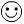# Trichotomy Property

The Trichotomy Property means that when you are comparing 2 numbers, one of the following must be true: the first number is bigger than the second number, the first number is smaller than the second number or the two numbers are equal. This is a common sense property with a hard nameLook how easy this is:

Example 1: given two numbers 5 and 7

• 5 is less than 7     5 < 7

• 5 is equal to 7       5 = 7

• 5 is greater than 7     5 > 7

• Which statement is true? 5 is less than 7.

Can more than one statement be true? No. 5 can not be equal to or greater than 7.

Example 2: Todd has 4 apples and Jorge has 10 apples. Which statement is true?

• Todd has more apples than Jorge.
• Todd has the same amount of apples as Jorge.
• Todd has fewer apples than Jorge.

Example 3: Amanda scored more points in basketball than Lauren. What else could we say about the scores?

• Lauren could NOT have scored more than Amanda
• Lauren could NOT have scored the same amount as Amanda.

Let's sum this up by using variables (letters instead of numbers). Only one statement can be true about any 2 numbers a and b.

a < b     a = b     or    a > b

One last example: Alex is shorter than Barry. It follows than Barry is taller than Alex and they cannot be the same height.
Related Links:
Math
algebra
Inverse Properties of Addition and Multiplication
Distributive Property

To link to this Trichotomy Property page, copy the following code to your site: